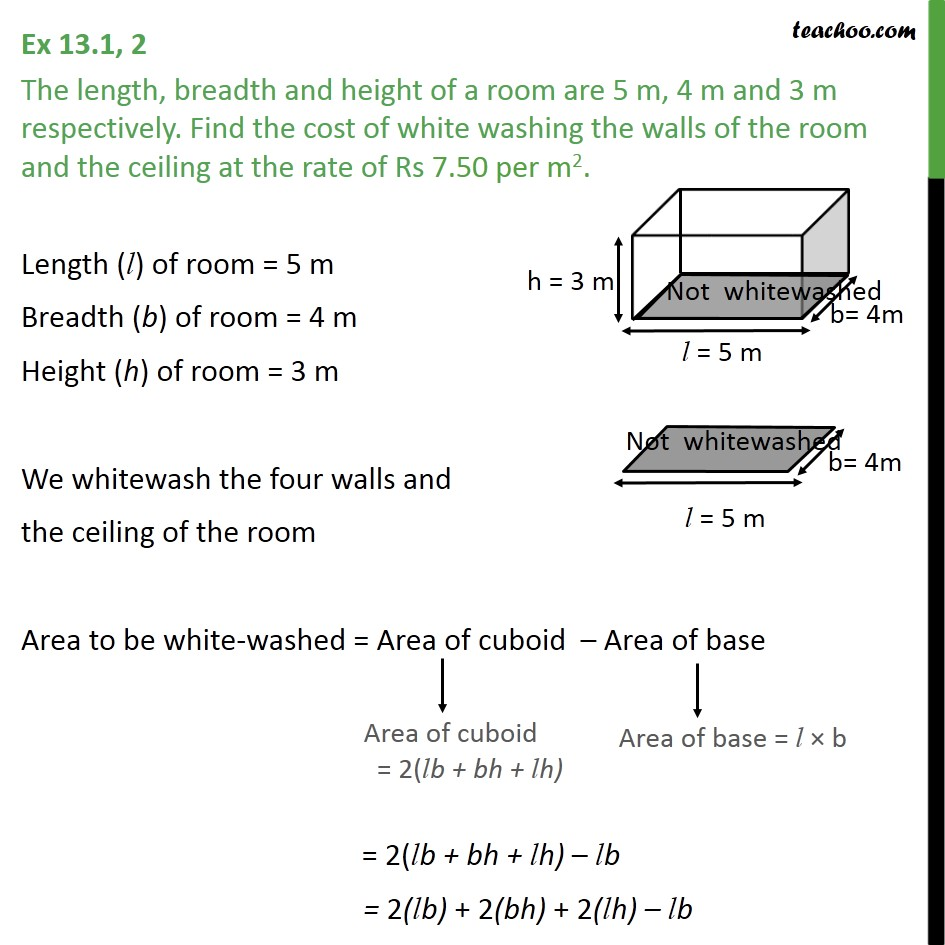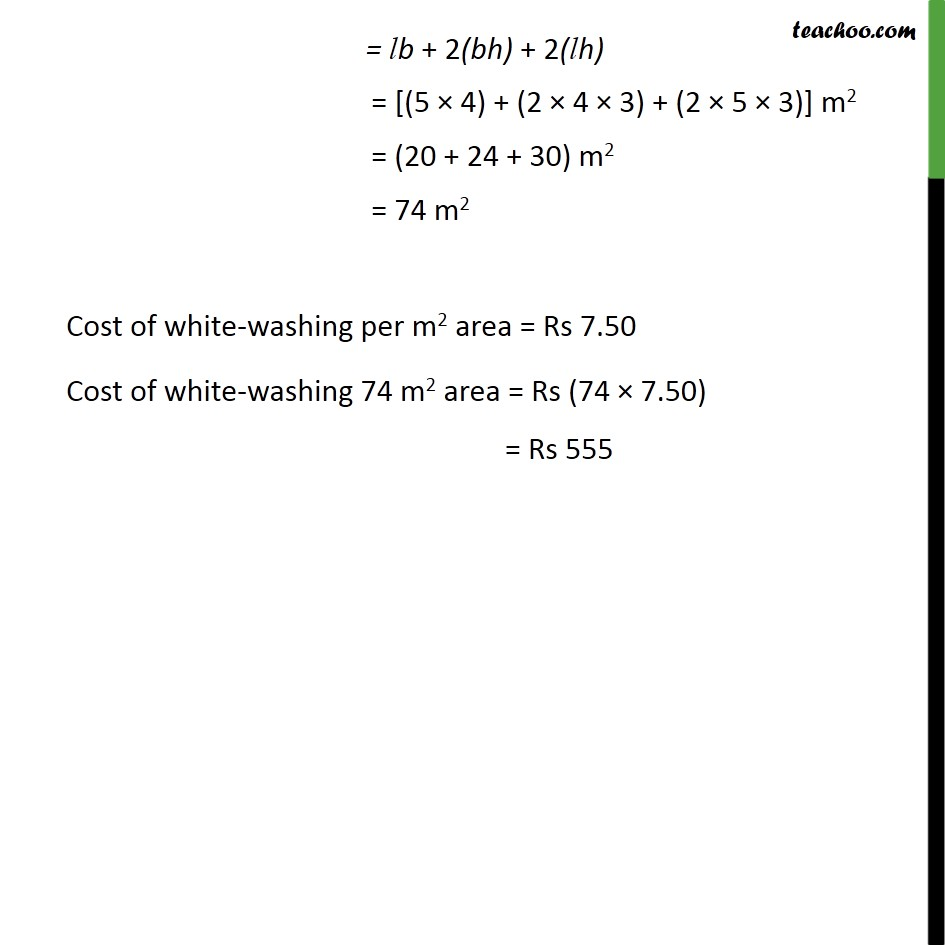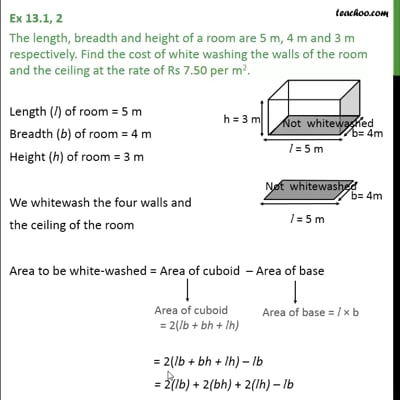Area Of Cube/ Cuboid

Chapter 13 Class 9 Surface Areas and Volumes
Concept wiseThis video is only available for Teachoo black users

Introducing your new favourite teacher - Teachoo Black, at only ₹83 per month

### Transcript

Ex 13.1, 2 The length, breadth and height of a room are 5 m, 4 m and 3 m respectively. Find the cost of white washing the walls of the room and the ceiling at the rate of Rs 7.50 per m2. Length (l) of room = 5 m Breadth (b) of room = 4 m Height (h) of room = 3 m We whitewash the four walls and the ceiling of the room Area to be white-washed = Area of cuboid – Area of base = 2(lb + bh + lh) – lb = 2(lb) + 2(bh) + 2(lh) – lb = lb + 2(bh) + 2(lh) = [(5 × 4) + (2 × 4 × 3) + (2 × 5 × 3)] m2 = (20 + 24 + 30) m2 = 74 m2 Cost of white-washing per m2 area = Rs 7.50 Cost of white-washing 74 m2 area = Rs (74 × 7.50)